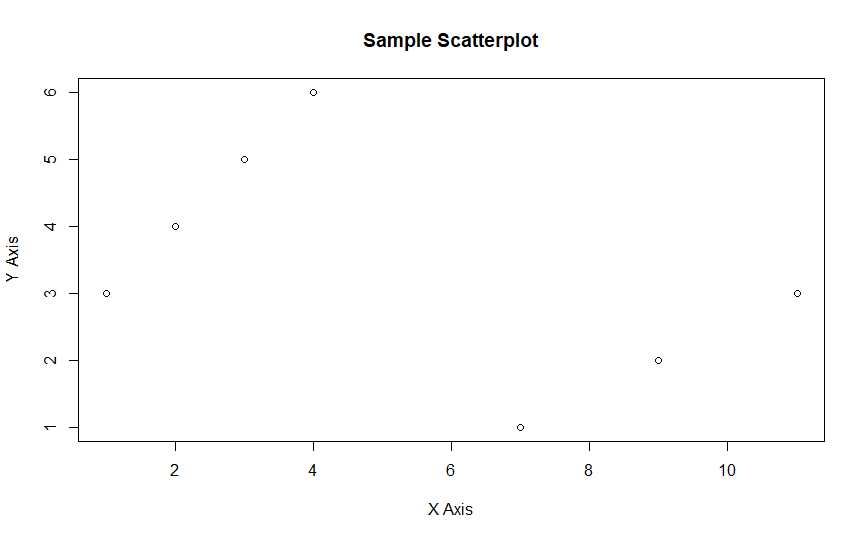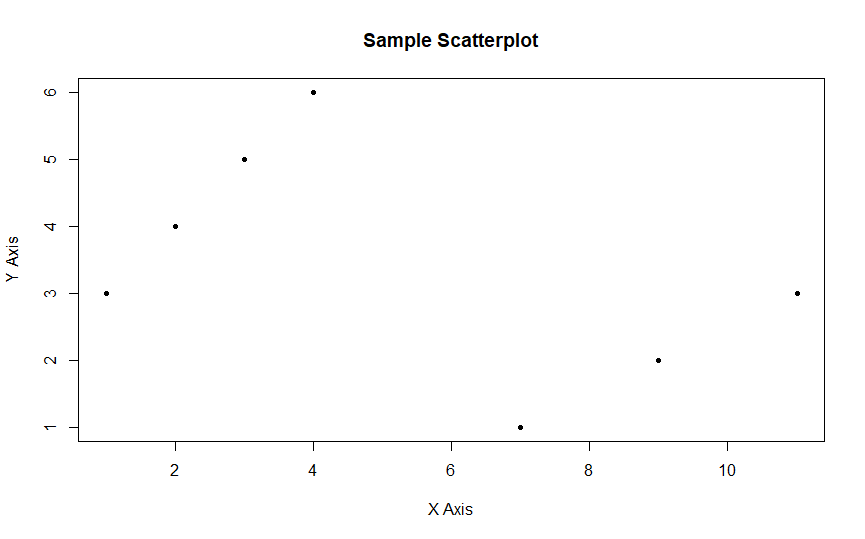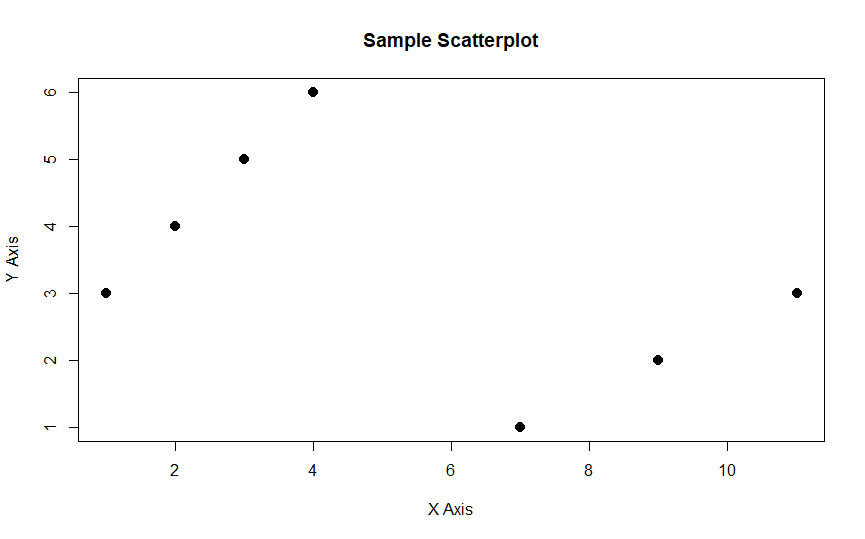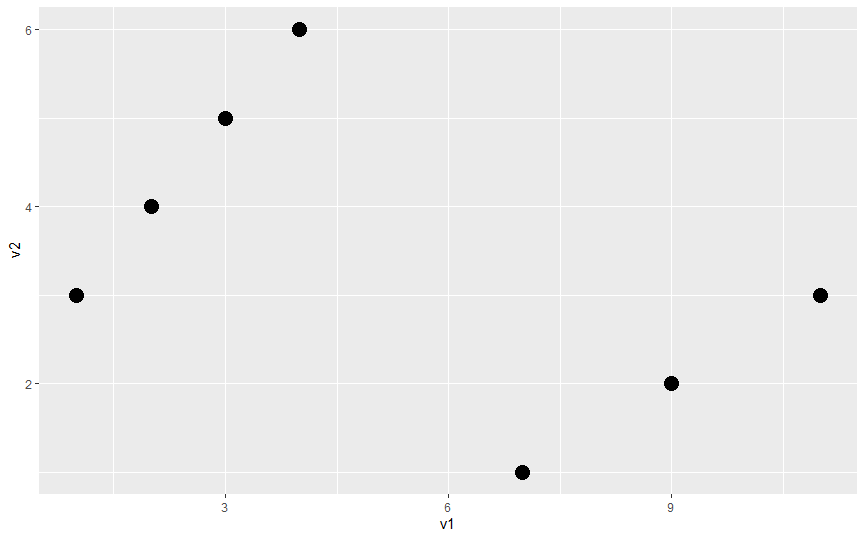# Change Point Size in Graphs in R

A scatterplot is one of the most basic and popularly-used plots in R. It simply plots a point depending on the value of two variables, each at the x and the y-axis, respectively. Scatterplots can help identify any underlying pattern between these variables and show how the values are related to each other.

The following example shows a simple scatterplot graph using the `plot()` function:

``````v1 <- c(1,2,3,4,11,9,7)
v2 <- c(3,4,5,6,3,2,1)
plot(x = v1, y = v2, xlab = "X Axis",ylab = "Y Axis",
main = "Sample Scatterplot")
``````Notice that we use the `xlab`, `ylab`, and `main` parameter to add the title and labels of X and Y axes.

We can set the size and the shape of the points using the `pch` and the `cex` parameters.

In R, we can have different symbols for a plot. We can have a simple empty circle, square, triangle, or filled shapes, and many more. We use the `pch` parameter to specify the shape of the point.

The `pch` value ranges from 1 to 25, corresponding to different shapes.

In the code below, we will set the `pch` to 20.

``````plot(x = v1, y = v2, xlab = "X Axis",ylab = "Y Axis",
main = "Sample Scatterplot", pch = 20)
``````We can use the `cex` argument to set the size of the points to make them more readable. This parameter is generally used with the `par` function to set other plotting parameters, but here we use it in the `plot` function as shown below.

``````plot(x = v1, y = v2, xlab = "X Axis",ylab = "Y Axis",
main = "Sample Scatterplot", pch = 20, cex = 2)
``````Notice the difference in the graph above and how the size of the points has increased.

In case you use the `qplot()` function to plot the scatter plot, we can set the size of the points using the `size` parameter. See the following code.

``````qplot(v1,v2, size= I(5))
``````The same parameter can also be applied while using the `ggplot()` function.